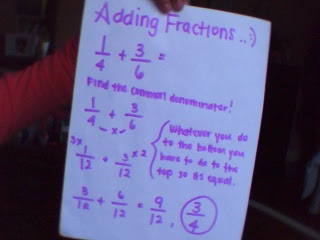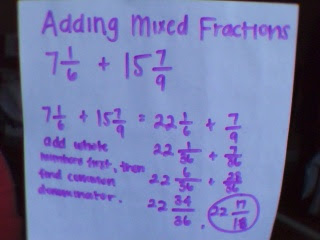### Carrie's Last Fraction Post

Monday, May 11, 2009
The first thing you have to do when adding fractions is to find the common denominator. Then you add together the numerators and add the denominators together. The last step is optional, you reduce or simplify your answer (the fraction) if you can.When you're adding mixed fractions you can add just the whole numbers right away or you can convert the mixed fraction into a improper fraction. Once you've done that you have to find the common denominator, and then add all the numerators together and the denominators together. If you converted your mixed fraction into a improper fraction for your first step, you will have an improper fraction as your answer. You shouldn't have an improper fraction as an answer, so convert it into a mixed fraction. The last step is to reduce or simply your answer if you can.Subtracting fractions. The first thing you're going to do is to find the common denominator. The second thing you have to do is to subtract all numerators together and all or the denominators together. And the last thing you have to do is to reduce your answer or simply it if you can.Multiplying fractions is the easiest! All you have to do is to multiply numerator by numerator and the denominator by denominator, and reduce your answer if you can.Multiplying mixed fractions is almost the same as multiplying proper fractions, except you have to convert the mixed fractions into improper fractions. You do the same thing, like in multiplying proper fractions where you multiply numerator by numerator and denominator by denominator. Then you have to simply reduce or simplify your answer and convert it into a mixed fraction.When you divide fractions you have to find the reciprocal (the reciprocal is the fraction fliped up-side-down, what makes it a whole number.) Then use multiplication, multiply the numerators and denominators together. Reduce if you can.The first thing you have to do when dividing mixed fraction is to convert any mixed fractions into improper fractions. Then do the same first 2 steps in the previous explanation, then you convert it into a mixed fraction. If you can, reduce or simplify your answer.I chose to do problem 2 & 3